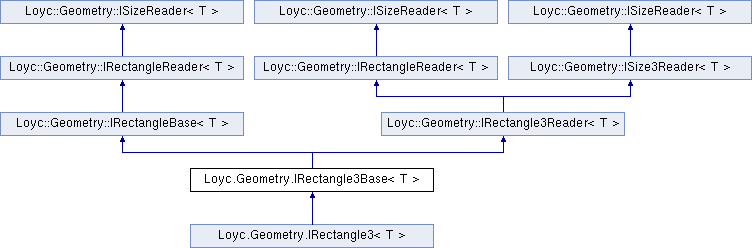Enhanced C# Language of your choice: library documentation
Loyc.Geometry.IRectangle3Base< T > Interface Template Reference

Represents a mutable 3D rectangular prism. More...

Source file:
Inheritance diagram for Loyc.Geometry.IRectangle3Base< T >:Remarks

Represents a mutable 3D rectangular prism.

Template Parameters
 T Type of each coordinate.

This interface can represent one of three types of rectangles: either "normal" rectangles with fundamental variables X, Y, Z, Width, Height, Depth, bounding rectangles with two coordinate ranges (X1-X2), (Y1-Y2), and (Z1-Z2), or pairs of points (X1, Y1, Z1) and (X2, Y2, Z2).

Because of this fact, it is not clear when if modify X1 whether this should affect X2 or Width. Similarly for Y1, Z1, X2, Y2 and Z2: should the size property or the opposite boundary change?. To resolve this question, this interface does not allow you to modify the coordinates individually; instead you must change them in pairs: you either set X and Width together, or X1 and X2 together; and similarly for Y1/Y2/Height and Z1/Z2/Depth.

You can also call the extension methods SetRect() or SetRange() to set all six coordinates at once.

IRectangle3<T>

Public Member Functions

void SetZAndDepth (T z, T depth)

void SetZRange (T z1, T z2)Public Member Functions inherited from Loyc.Geometry.IRectangleBase< T >
void SetXAndWidth (T x, T width)

void SetYAndHeight (T y, T height)

void SetXRange (T x1, T x2)

void SetYRange (T x1, T x2)Properties inherited from Loyc.Geometry.IRectangleReader< T >
X1 [get]

Y1 [get]

X2 [get]

Y2 [get]Properties inherited from Loyc.Geometry.ISizeReader< T >
Width [get]
Gets the width of a rectangle (the difference between X coordinates). More...

Height [get]
Gets the height of a rectangle (the difference between Y coordinates). More...Properties inherited from Loyc.Geometry.IRectangle3Reader< T >
Z1 [get]

Z2 [get]Properties inherited from Loyc.Geometry.ISize3Reader< T >
Depth [get]
Gets the depth of a rectangle (the difference between Z coordinates). More...